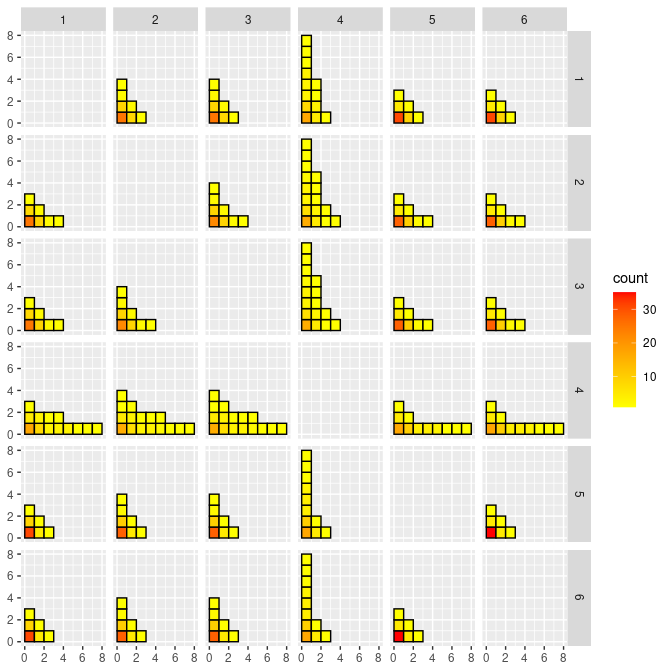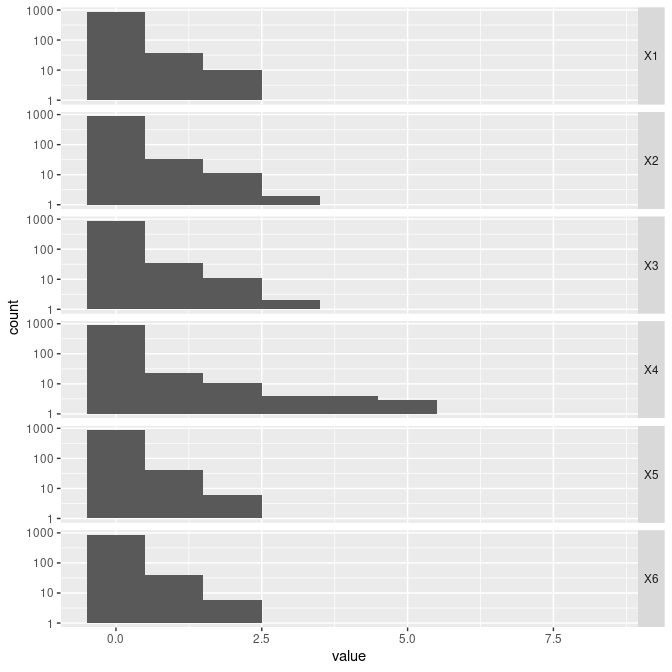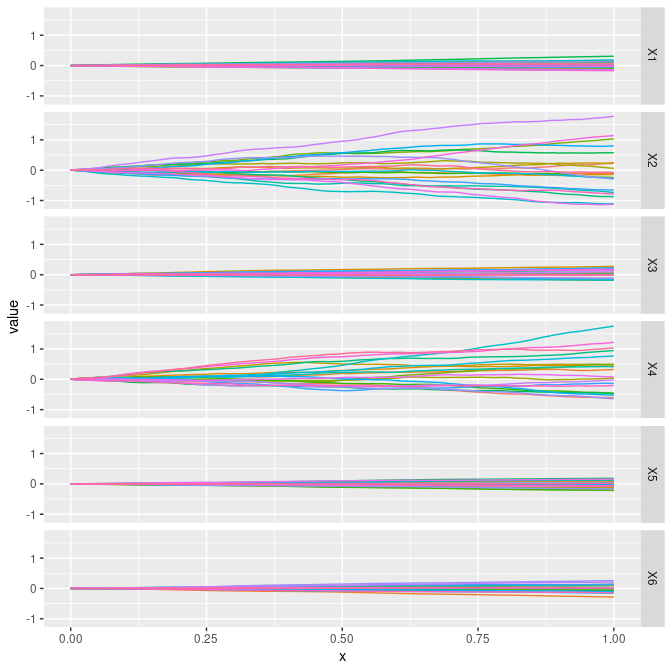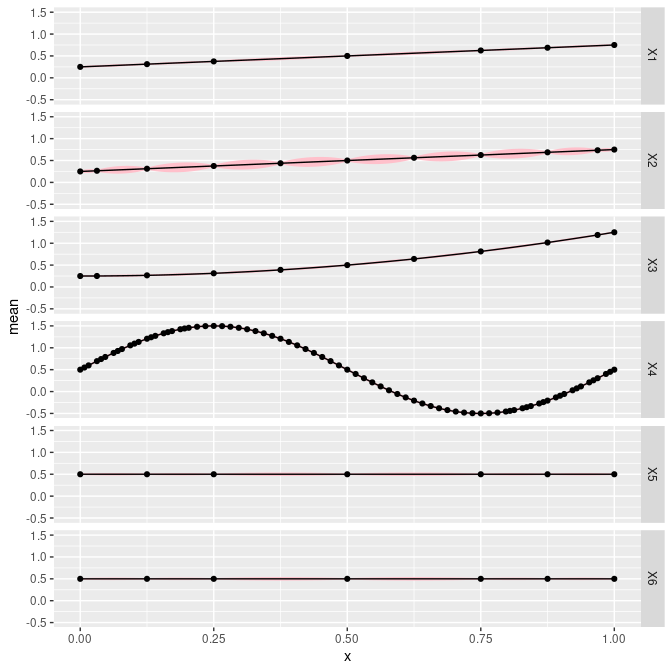# An Introduction to the CGGP Package

## Introduction

The R package CGGP implements the adaptive composite grid using Gaussian process models presented in a forthcoming publication by Matthew Plumlee, Collin Erickson, Bruce Ankenman, et al. It provides an algorithm for running sequential computer experiments with thousands of data points.

The composite grid structure imposes strict requirements on which points should be evaluated. The inputs chosen to be evaluated are specified by the algorithm. This does not work with preexisting data sets, it is not a regression technique. This only works in sequential experimental design scenarios: you start with no data, and then decide which points to evaluate in batches according to the algorithm.

When to use it:

• You have not started collecting data yet, or only have a small data set

• You will collect 5,000 to 100,000 data points

• You will collect data iteratively

• Your simulation has four to twenty input dimensions

• The simulation output is deterministic (evaluating the same input twice always returns the same value)

Why you should use it:

• Gaussian process models, a common model choice for modeling computer experiments, have computation issues for more than a thousand data points

• Accuracy is much better than popular machine learning algorithms, which are better suited for noisy data

## How to use CGGP

You should have a deterministic function that takes $$d$$-dimensional input that you can evaluate for any point in the unit cube $$[0,1]^d$$. The points generated by the algorithm will be given to you to evaluate, then you will return the function output for each input point. The model will be fit to this data and you will be able to use it to make predictions or evaluate additional points.

To begin, use CGGPcreate to create a CGGP object. You must tell it the number of input dimensions $$d$$ and the number of points for the first batch. For example, if $$d=6$$ and you want to begin will 100 points, you can create a model mod with the following code.

library(CGGP)
# Create the initial design
d <- 6
mod <- CGGPcreate(d=d, batchsize=100)
mod
#> CGGP object
#>    d = 6
#>    output dimensions = 1
#>    CorrFunc = PowerExponential
#>    number of design points             = 97
#>    number of unevaluated design points = 97
#>    Available functions:
#>      - CGGPfit(CGGP, Y) to update parameters with new data
#>      - CGGPpred(CGGP, xp) to predict at new points
#>      - CGGPappend(CGGP, batchsize) to add new design points
#>      - CGGPplot<name>(CGGP) to visualize CGGP model

Now mod will contain all the relevant information for the composite grid design and model. Most importantly, it has the initial set of points that must be evaluated. These are accessed as mod$design. str(mod$design)
#>  num [1:97, 1:6] 0.5 0.125 0.875 0.5 0.5 0.5 0.5 0.5 0.5 0.5 ...

Now you must pass these points to your function to be evaluated.

f <- function(x){x*x + x^2 + (x+.5)*sin(2*pi*x)}
Y <- apply(mod$design, 1, f) Once you have the data for each row of mod$design, you can now fit the model using CGGPfit.

mod <- CGGPfit(mod, Y)
mod
#> CGGP object
#>    d = 6
#>    output dimensions = 1
#>    CorrFunc = PowerExponential
#>    number of design points             = 97
#>    number of unevaluated design points = 0
#>    Available functions:
#>      - CGGPfit(CGGP, Y) to update parameters with new data
#>      - CGGPpred(CGGP, xp) to predict at new points
#>      - CGGPappend(CGGP, batchsize) to add new design points
#>      - CGGPplot<name>(CGGP) to visualize CGGP model

Now that you have a fitted model, you can either use it to make predictions at points, or augment the design with additional runs.

To use the model to predict the output at new points, use the function CGGPpred or predict. Letxp be the matrix whose rows are the points that you want to make predictions at. Then the following will return a list with the mean and predictive variance for each row of xp.

xp <- matrix(runif(d*100), ncol=d)
str(CGGPpred(CGGP=mod, xp=xp))
#> List of 2
#>  $mean: num [1:100, 1] 1.835 1.859 -0.808 0.527 -0.555 ... #>$ var : num [1:100, 1] 0.02241 0.01857 0.01117 0.00431 0.02726 ...

To add points to the design, use the function CGGPappend, and include how many points you want to add. This is the maximum number; it may append a smaller number of points if it is not able to reach the specified number. To add 200 points:

mod <- CGGPappend(mod, 200, "MAP")
mod
#> CGGP object
#>    d = 6
#>    output dimensions = 1
#>    CorrFunc = PowerExponential
#>    number of design points             = 297
#>    number of unevaluated design points = 200
#>    Available functions:
#>      - CGGPfit(CGGP, Y) to update parameters with new data
#>      - CGGPpred(CGGP, xp) to predict at new points
#>      - CGGPappend(CGGP, batchsize) to add new design points
#>      - CGGPplot<name>(CGGP) to visualize CGGP model

You would choose to add points to the design in multiple steps, as opposed to all in a single step, so that the fitted model can be used to efficiently select the points to augment the design.

Once you have appended new points, you need to evaluate them and fit the model again. You can access the new design points that need to be evaluated using mod$design_unevaluated. Ynew <- apply(mod$design_unevaluated, 1, f)
mod <- CGGPfit(mod, Ynew=Ynew)
mod
#> CGGP object
#>    d = 6
#>    output dimensions = 1
#>    CorrFunc = PowerExponential
#>    number of design points             = 297
#>    number of unevaluated design points = 0
#>    Available functions:
#>      - CGGPfit(CGGP, Y) to update parameters with new data
#>      - CGGPpred(CGGP, xp) to predict at new points
#>      - CGGPappend(CGGP, batchsize) to add new design points
#>      - CGGPplot<name>(CGGP) to visualize CGGP model

### Plotting CGGP objects

It is very difficult to comprehend what designs in high dimensions look like, but we would like to be able to have visuals and diagnostics to make sure the design is sensible and to try to get an idea of what it is doing. We have implemented a few plotting functions in the CGGP package that aim to provide a visualization of the design and its parameters.

The function CGGPplotblocks can be used to view the blocks (indexes) when projected down to each pair of dimensions. Dimensions that are more interesting should have a wider variety in values.

CGGPplotblocks(mod)Histograms for the values of each index for each dimension are given by CGGPplothist. Dimensions with more spread to the right can be thought of as having been allocated more simulation effort.

CGGPplothist(mod)
#> Warning: Transformation introduced infinite values in continuous y-axis
#> Warning: Removed 23 rows containing missing values (geom_bar).The correlation parameters for each input dimension can also provide useful information about how active each dimension is.

CGGPplotcorr shows Gaussian process (GP) samples using the correlation parameters for each input dimension. The lines shown do not depict each dimension, but give an idea of what GP models with the same correlation parameters look like.

CGGPplotcorr(mod)The function CGGPplotslice shows how the output changes when a single input is varied across its range from 0 to 1 while holding all the other inputs at a constant value. This plot may also be referred to as a slice plot. By default the other input values are held constant at 0.5, but this can be changed with a parameter. The dots on the plot are included for points that were measured and used to fit the model. These dots generally only appear when the other dimensions are held at 0.5.

CGGPplotslice(mod)